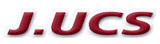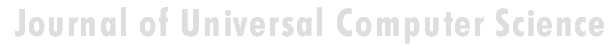Search
 Submission Procedure share: |available in:   PDF (215 kB) PS (49 kB)

get:
 Similar Docs BibTeX Write a comment
get:

DOI:   10.3217/jucs-001-07-0469

Round-off Error Propagation in the Solution of the Heat Equation by finite Differences

Fabienne Jézéquel (Université Pierre et Marie Curie, France)

Abstract: The effect of round-off errors on the numerical solution of the heat equation by finite differences can be theoretically determined by computing the mean error at each time step. The floating point error propagation is then theoretically time linear. The experimental simulations agree with this result for the towards zero rounding arithmetic. However the results are not so good for the rounding to the nearest artihmetic. The theoretical formulas provide an approximation of the experimental round-off errors. In these formulas the mean value of the assignment operator is used, and consequently, their reliability depends on the arithmetic used.

Keywords: Floating point arithmetic, finite difference methods, numerical error propagation, partial differential equations

Categories: G.1.8